Showing posts with label Albert Einstein. Show all posts
Showing posts with label Albert Einstein. Show all posts

## Thursday, February 27, 2014

### What is the Total Field?

The general theory of relativity is as yet incomplete insofar as it has been able to apply the general principle of relativity satisfactorily only to gravitational fields, but not to the total field. We do not yet know with certainty by what mathematical mechanism the total field in space is to be described and what the general invariant laws are to which this total field is subject. One thing, however, seems certain: namely, that the general principal of relativity will prove a necessary and effective tool for the solution of the problem for the total field. -Out of My Later Years, Pg 48, Albert Einstein (bold and underlined added for emphasis)

***

You see, I am formulating qualifiers as to the nature of the question about the total field? The idea here is that from a symmetrical state,  and not wanting infinity to be such a thing,  what is the underlying the question of what exists as a moduli figure? The total field is as if,  some vacua, which has a hold on the idea of time and where this leads one too? No time.

So there is then this evolution of the moduli figure that rests in the valley, as to the question of what as a probabilistic event may be determined,  as if,  the higg's. It reveals that the higg's,  is not the end all too the story and I question the nature of the total field..

## Wednesday, February 19, 2014

### Albert Einstein and Rabindranath TagoreRabindranath with Einstein in 1930

Was reading a interesting article called, " When Einstein Met Tagore.  by
On July 14, 1930, Albert Einstein welcomed into his home on the outskirts of Berlin the Indian philosopher Rabindranath Tagore. The two proceeded to have one of the most stimulating, intellectually riveting conversations in history, exploring the age-old friction between science and religion. Science and the Indian Tradition: When Einstein Met Tagore recounts the historic encounter, amidst a broader discussion of the intellectual renaissance that swept India in the early twentieth century, germinating a curious osmosis of Indian traditions and secular Western scientific doctrine.

***

## Tuesday, June 11, 2013

### Time Begins, When Counting Begins?

Like Copernicus' heliocentric theory, Newton's law of gravitation, and Darwin's theory of evolution, non-Euclidean geometry has radically affected science, philosophy, and religion. It is fair to say that no more cataclysmic event has ever taken place in the history of all thought.Saccheri's Flaw while eliminating Euclid's "Flaw" The Evolution of Non-Euclidean Geometry
The basis of any experience has it's counter part in how we have established the lines to which we place all experiencing on? You cannot count backward to zero(what is before zero...ummmmmm nothing), so what takes zero's place? It would be like asking what existed before this universe, so fundamentally they looked at issues around the false vacuum to the true. But cosmologically they call this universe "a box," and anything outside of it not fundamental?

Time has no independent existence apart from the order of events by which we measure it.Albert Einstein

When does counting begin? Discover Patterns.  Fibonacci Numbers perhaps? How does that apply to the natural world?

Any measure then, serves to activate a counting to begin? So you choose to be discrete. Some how you cannot distance yourself from any operation as to say the location is other then a configuration space, and that you are operating within it?

So the question is, when do you first become aware? What is considered outside of time, if you think that time refers too, when counting begins? So you are in your subjective states, whether these are real or not remains to be seen, so how do you quantify this? Do you have a way of keeping time in the subjective world.

Abstract space(mathematics) are totally outside of time?

I guess it is sort of like asking what first cause is to imply. Yet, theoretical definition is to say that string theory pushes back time much further to such a beginning then Steven Weinberg's first three minutes. The act in itself is related to "microseconds" when pushing back perspective, and not Weinberg's minutes

The background(WMAP) initially is a foundation with which the universe is painted. Then you add in the progressiveness of the parameters with which you use to define the universe?

So theoretically, you start counting when? The abstractness is contained in the mathematical structure of the universe which has been chosen to be perceived by observing in that abstract framework.

When you've chosen virtually reality, you have choose to model the framework(subjective /objectively) as well? We use it to model abstract language. Is that real?

So recap on use of measure of natural units then.

In physics, natural units are physical units of measurement defined in terms of universal physical constants in such a manner that some chosen physical constants take on the numerical value of one when expressed in terms of a particular set of natural units. Natural units are intended to elegantly simplify particular algebraic expressions appearing in physical law or to normalize some chosen physical quantities that are properties of universal elementary particles and that may be reasonably believed to be constant. However, what may be believed and forced to be constant in one system of natural units can very well be allowed or even assumed to vary in another natural unit system. Natural units are natural because the origin of their definition comes only from properties of nature and not from any human construct. Planck units are often, without qualification, called "natural units" but are only one system of natural units among other systems. Planck units might be considered unique in that the set of units are not based on properties of any prototype, object, or particle but are based only on properties of free space.Natural units
So we have effectively run into a problem.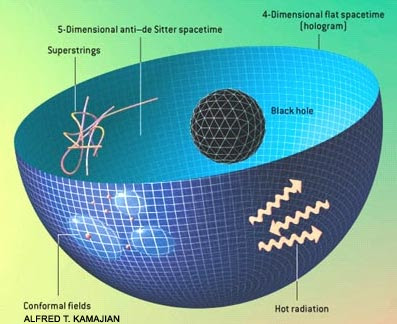TWO UNIVERSES of different dimension and obeying disparate physical laws are rendered completely equivalent by the holographic principle. Theorists have demonstrated this principle mathematically for a specific type of five-dimensional spacetime ("anti–de Sitter") and its four-dimensional boundary. In effect, the 5-D universe is recorded like a hologram on the 4-D surface at its periphery. Superstring theory rules in the 5-D spacetime, but a so-called conformal field theory of point particles operates on the 4-D hologram. A black hole in the 5-D spacetime is equivalent to hot radiation on the hologram--for example, the hole and the radiation have the same entropy even though the physical origin of the entropy is completely different for each case. Although these two descriptions of the universe seem utterly unalike, no experiment could distinguish between them, even in principle.

When you are looking out toward the universe you are looking for the reasons as to why the universe is doing what it is doing. What is happening in one place in terms of black hole production in the cosmos? Do these have implications, as in other cosmological sources as to imply, the universe is doing what it is doing?

## Sunday, April 17, 2011

### Space

Space is the boundless, three-dimensional extent in which objects and events occur and have relative position and direction. Physical space is often conceived in three linear dimensions, although modern physicists usually consider it, with time, to be part of the boundless four-dimensional continuum known as spacetime. In mathematics one examines 'spaces' with different numbers of dimensions and with different underlying structures. The concept of space is considered to be of fundamental importance to an understanding of the physical universe although disagreement continues between philosophers over whether it is itself an entity, a relationship between entities, or part of a conceptual framework.

Debates concerning the nature, essence and the mode of existence of space date back to antiquity; namely, to treatises like the Timaeus of Plato, in his reflections on what the Greeks called: chora / Khora (i.e. 'space'), or in the Physics of Aristotle (Book IV, Delta) in the definition of topos (i.e. place), or even in the later 'geometrical conception of place' as 'space qua extension' in the Discourse on Place (Qawl fi al-makan) of the 11th century Arab polymath Ibn al-Haytham (Alhazen). Many of these classical philosophical questions were discussed in the Renaissance and then reformulated in the 17th century, particularly during the early development of classical mechanics. In Isaac Newton's view, space was absolute - in the sense that it existed permanently and independently of whether there were any matter in the space.

Other natural philosophers, notably Gottfried Leibniz, thought instead that space was a collection of relations between objects, given by their distance and direction from one another. In the 18th century, the philosopher and theologian George Berkely attempted to refute the 'visibility of spatial depth' in his Essay Towards a New Theory of Vision. Later, the great metaphysician Immanuel Kant described space and time as elements of a systematic framework that humans use to structure their experience; he referred to 'space' in his Critique of Pure Reason as being: a subjective 'pure a priori form of intuition', hence that its existence depends on our human faculties.

In the 19th and 20th centuries mathematicians began to examine non-Euclidean geometries, in which space can be said to be curved, rather than flat. According to Albert Einstein's theory of general relativity, space around gravitational fields deviates from Euclidean space. Experimental tests of general relativity have confirmed that non-Euclidean space provides a better model for the shape of space.

## Philosophy of space

### Leibniz and Newton

In the seventeenth century, the philosophy of space and time emerged as a central issue in epistemology and metaphysics. At its heart, Gottfried Leibniz, the German philosopher-mathematician, and Isaac Newton, the English physicist-mathematician, set out two opposing theories of what space is. Rather than being an entity that independently exists over and above other matter, Leibniz held that space is no more than the collection of spatial relations between objects in the world: "space is that which results from places taken together". Unoccupied regions are those that could have objects in them, and thus spatial relations with other places. For Leibniz, then, space was an idealised abstraction from the relations between individual entities or their possible locations and therefore could not be continuous but must be discrete. Space could be thought of in a similar way to the relations between family members. Although people in the family are related to one another, the relations do not exist independently of the people. Leibniz argued that space could not exist independently of objects in the world because that implies a difference between two universes exactly alike except for the location of the material world in each universe. But since there would be no observational way of telling these universes apart then, according to the identity of indiscernibles, there would be no real difference between them. According to the principle of sufficient reason, any theory of space that implied that there could be these two possible universes, must therefore be wrong.

Newton took space to be more than relations between material objects and based his position on observation and experimentation. For a relationist there can be no real difference between inertial motion, in which the object travels with constant velocity, and non-inertial motion, in which the velocity changes with time, since all spatial measurements are relative to other objects and their motions. But Newton argued that since non-inertial motion generates forces, it must be absolute. He used the example of water in a spinning bucket to demonstrate his argument. Water in a bucket is hung from a rope and set to spin, starts with a flat surface. After a while, as the bucket continues to spin, the surface of the water becomes concave. If the bucket's spinning is stopped then the surface of the water remains concave as it continues to spin. The concave surface is therefore apparently not the result of relative motion between the bucket and the water. Instead, Newton argued, it must be a result of non-inertial motion relative to space itself. For several centuries the bucket argument was decisive in showing that space must exist independently of matter.

### Kant

In the eighteenth century the German philosopher Immanuel Kant developed a theory of knowledge in which knowledge about space can be both a priori and synthetic. According to Kant, knowledge about space is synthetic, in that statements about space are not simply true by virtue of the meaning of the words in the statement. In his work, Kant rejected the view that space must be either a substance or relation. Instead he came to the conclusion that space and time are not discovered by humans to be objective features of the world, but are part of an unavoidable systematic framework for organizing our experiences.

### Non-Euclidean geometry

Euclid's Elements contained five postulates that form the basis for Euclidean geometry. One of these, the parallel postulate has been the subject of debate among mathematicians for many centuries. It states that on any plane on which there is a straight line L1 and a point P not on L1, there is only one straight line L2 on the plane that passes through the point P and is parallel to the straight line L1. Until the 19th century, few doubted the truth of the postulate; instead debate centered over whether it was necessary as an axiom, or whether it was a theory that could be derived from the other axioms. Around 1830 though, the Hungarian János Bolyai and the Russian Nikolai Ivanovich Lobachevsky separately published treatises on a type of geometry that does not include the parallel postulate, called hyperbolic geometry. In this geometry, an infinite number of parallel lines pass through the point P. Consequently the sum of angles in a triangle is less than 180o and the ratio of a circle's circumference to its diameter is greater than pi. In the 1850s, Bernhard Riemann developed an equivalent theory of elliptical geometry, in which no parallel lines pass through P. In this geometry, triangles have more than 180o and circles have a ratio of circumference-to-diameter that is less than pi.
Type of geometry Number of parallels Sum of angles in a triangle Ratio of circumference to diameter of circle Measure of curvature
Hyperbolic Infinite < 180o > π < 0
Euclidean 1 180o π 0
Elliptical 0 > 180o < π > 0

### Gauss and Poincaré

Although there was a prevailing Kantian consensus at the time, once non-Euclidean geometries had been formalised, some began to wonder whether or not physical space is curved. Carl Friedrich Gauss, a German mathematician, was the first to consider an empirical investigation of the geometrical structure of space. He thought of making a test of the sum of the angles of an enormous stellar triangle and there are reports he actually carried out a test, on a small scale, by triangulating mountain tops in Germany.

Henri Poincaré, a French mathematician and physicist of the late 19th century introduced an important insight in which he attempted to demonstrate the futility of any attempt to discover which geometry applies to space by experiment. He considered the predicament that would face scientists if they were confined to the surface of an imaginary large sphere with particular properties, known as a sphere-world. In this world, the temperature is taken to vary in such a way that all objects expand and contract in similar proportions in different places on the sphere. With a suitable falloff in temperature, if the scientists try to use measuring rods to determine the sum of the angles in a triangle, they can be deceived into thinking that they inhabit a plane, rather than a spherical surface. In fact, the scientists cannot in principle determine whether they inhabit a plane or sphere and, Poincaré argued, the same is true for the debate over whether real space is Euclidean or not. For him, which geometry was used to describe space, was a matter of convention. Since Euclidean geometry is simpler than non-Euclidean geometry, he assumed the former would always be used to describe the 'true' geometry of the world.

### Einstein

In 1905, Albert Einstein published a paper on a special theory of relativity, in which he proposed that space and time be combined into a single construct known as spacetime. In this theory, the speed of light in a vacuum is the same for all observers—which has the result that two events that appear simultaneous to one particular observer will not be simultaneous to another observer if the observers are moving with respect to one another. Moreover, an observer will measure a moving clock to tick more slowly than one that is stationary with respect to them; and objects are measured to be shortened in the direction that they are moving with respect to the observer.

Over the following ten years Einstein worked on a general theory of relativity, which is a theory of how gravity interacts with spacetime. Instead of viewing gravity as a force field acting in spacetime, Einstein suggested that it modifies the geometric structure of spacetime itself. According to the general theory, time goes more slowly at places with lower gravitational potentials and rays of light bend in the presence of a gravitational field. Scientists have studied the behaviour of binary pulsars, confirming the predictions of Einstein's theories and Non-Euclidean geometry is usually used to describe spacetime.

## Mathematics

In modern mathematics spaces are defined as sets with some added structure. They are frequently described as different types of manifolds, which are spaces that locally approximate to Euclidean space, and where the properties are defined largely on local connectedness of points that lie on the manifold. There are however, many diverse mathematical objects that are called spaces. For example, vector spaces such as function spaces may have infinite numbers of independent dimensions and a notion of distance very different to Euclidean space, and topological spaces replace the concept of distance with a more abstract idea of nearness.

## Physics

### Classical mechanics

Classical mechanics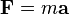$\mathbf{F} = m \mathbf{a}$
Newton's Second Law
History of classical mechanics · Timeline of classical mechanics
[hide]Fundamental concepts
Space · Time · Mass · D'Alembert's principle
v · d · e
Space is one of the few fundamental quantities in physics, meaning that it cannot be defined via other quantities because nothing more fundamental is known at the present. On the other hand, it can be related to other fundamental quantities. Thus, similar to other fundamental quantities (like time and mass), space can be explored via measurement and experiment.

### Astronomy

Astronomy is the science involved with the observation, explanation and measuring of objects in outer space.

### Relativity

Before Einstein's work on relativistic physics, time and space were viewed as independent dimensions. Einstein's discoveries showed that due to relativity of motion our space and time can be mathematically combined into one object — spacetime. It turns out that distances in space or in time separately are not invariant with respect to Lorentz coordinate transformations, but distances in Minkowski space-time along space-time intervals are—which justifies the name.
In addition, time and space dimensions should not be viewed as exactly equivalent in Minkowski space-time. One can freely move in space but not in time. Thus, time and space coordinates are treated differently both in special relativity (where time is sometimes considered an imaginary coordinate) and in general relativity (where different signs are assigned to time and space components of spacetime metric).

Furthermore, in Einstein's general theory of relativity, it is postulated that space-time is geometrically distorted- curved -near to gravitationally significant masses.

Experiments are ongoing to attempt to directly measure gravitational waves. This is essentially solutions to the equations of general relativity, which describe moving ripples of spacetime. Indirect evidence for this has been found in the motions of the Hulse-Taylor binary system.

### Cosmology

Relativity theory leads to the cosmological question of what shape the universe is, and where space came from. It appears that space was created in the Big Bang, 13.7 billion years ago and has been expanding ever since. The overall shape of space is not known, but space is known to be expanding very rapidly due to the Cosmic Inflation.

## Spatial measurement

The measurement of physical space has long been important. Although earlier societies had developed measuring systems, the International System of Units, (SI), is now the most common system of units used in the measuring of space, and is almost universally used.

Currently, the standard space interval, called a standard meter or simply meter, is defined as the distance traveled by light in a vacuum during a time interval of exactly 1/299,792,458 of a second. This definition coupled with present definition of the second is based on the special theory of relativity in which the speed of light plays the role of a fundamental constant of nature.

## Geographical space

Geography is the branch of science concerned with identifying and describing the Earth, utilizing spatial awareness to try and understand why things exist in specific locations. Cartography is the mapping of spaces to allow better navigation, for visualization purposes and to act as a locational device. Geostatistics apply statistical concepts to collected spatial data to create an estimate for unobserved phenomena.

Geographical space is often considered as land, and can have a relation to ownership usage (in which space is seen as property or territory). While some cultures assert the rights of the individual in terms of ownership, other cultures will identify with a communal approach to land ownership, while still other cultures such as Australian Aboriginals, rather than asserting ownership rights to land, invert the relationship and consider that they are in fact owned by the land. Spatial planning is a method of regulating the use of space at land-level, with decisions made at regional, national and international levels. Space can also impact on human and cultural behavior, being an important factor in architecture, where it will impact on the design of buildings and structures, and on farming.

Ownership of space is not restricted to land. Ownership of airspace and of waters is decided internationally. Other forms of ownership have been recently asserted to other spaces — for example to the radio bands of the electromagnetic spectrum or to cyberspace.

Public space is a term used to define areas of land as collectively owned by the community, and managed in their name by delegated bodies; such spaces are open to all. While private property is the land culturally owned by an individual or company, for their own use and pleasure.

Abstract space is a term used in geography to refer to a hypothetical space characterized by complete homogeneity. When modeling activity or behavior, it is a conceptual tool used to limit extraneous variables such as terrain.

## In psychology

Psychologists first began to study the way space is perceived in the middle of the 19th century. Those now concerned with such studies regard it as a distinct branch of psychology. Psychologists analyzing the perception of space are concerned with how recognition of an object's physical appearance or its interactions are perceived.

Other, more specialized topics studied include amodal perception and object permanence. The perception of surroundings is important due to its necessary relevance to survival, especially with regards to hunting and self preservation as well as simply one's idea of personal space.

Several space-related phobias have been identified, including agoraphobia (the fear of open spaces), astrophobia (the fear of celestial space) and claustrophobia (the fear of enclosed spaces).

## Thursday, December 09, 2010

### Time Dilation

....... is a phenomenon (or two phenomena, as mentioned below) described by the theory of relativity. It can be illustrated by supposing that two observers are in motion relative to each other, or differently situated with regard to nearby gravitational masses. They each carry a clock of identical construction and function. Then, the point of view of each observer will generally be that the other observer's clock is in error (has changed its rate).

Both causes (distance to gravitational mass and relative speed) can operate together.

## Overview

Time dilation can arise from:
1. the relative velocity of motion between two observers, or
2. the difference in their distance from a gravitational mass.

### Relative velocity time dilation

When two observers are in relative uniform motion and far away from any gravitational mass, the point of view of each will be that the other's (moving) clock is ticking at a slower rate than the local clock. The faster the relative velocity, the greater the magnitude of time dilation. This case is sometimes called special relativistic time dilation. It is often interpreted as time "slowing down" for the other (moving) clock. But that is only true from the physical point of view of the local observer, and of others at relative rest (i.e. in the local observer's frame of reference). The point of view of the other observer will be that again the local clock (this time the other clock) is correct and it is the distant moving one that is slow. From a local perspective, time registered by clocks that are at rest with respect to the local frame of reference (and far from any gravitational mass) always appears to pass at the same rate.

### Gravitational time dilation

There is another case of time dilation, where both observers are differently situated in their distance from a significant gravitational mass, such as (for terrestrial observers) the Earth or the Sun. One may suppose for simplicity that the observers are at relative rest (which is not the case of two observers both rotating with the Earth—an extra factor described below). In the simplified case, the general theory of relativity describes how, for both observers, the clock that is closer to the gravitational mass, i.e. deeper in its "gravity well", appears to go slower than the clock that is more distant from the mass (or higher in altitude away from the center of the gravitational mass). That does not mean that the two observers fully agree: each still makes the local clock to be correct; the observer more distant from the mass (higher in altitude) measures the other clock (closer to the mass, lower in altitude) to be slower than the local correct rate, and the observer situated closer to the mass (lower in altitude) measures the other clock (farther from the mass, higher in altitude) to be faster than the local correct rate. They agree at least that the clock nearer the mass is slower in rate and on the ratio of the difference.

### Time dilation: special vs. general theories of relativity

In Albert Einstein's theories of relativity, time dilation in these two circumstances can be summarized:
• In special relativity (or, hypothetically far from all gravitational mass), clocks that are moving with respect to an inertial system of observation are measured to be running slower. This effect is described precisely by the Lorentz transformation.
Thus, in special relativity, the time dilation effect is reciprocal: as observed from the point of view of either of two clocks which are in motion with respect to each other, it will be the other clock that is time dilated. (This presumes that the relative motion of both parties is uniform; that is, they do not accelerate with respect to one another during the course of the observations.)

In contrast, gravitational time dilation (as treated in general relativity) is not reciprocal: an observer at the top of a tower will observe that clocks at ground level tick slower, and observers on the ground will agree about that, i.e. about the direction and the ratio of the difference. There is not full agreement, all the observers make their own local clocks out to be correct, but the direction and ratio of gravitational time dilation is agreed by all observers, independent of their altitude.

## Simple inference of time dilation due to relative velocity

Time dilation can be inferred from the observed fact of the constancy of the speed of light in all reference frames.    

This constancy of the speed of light means, counter to intuition, that speeds of material objects and light are not additive. It is not possible to make the speed of light appear faster by approaching at speed towards the material source that is emitting light. It is not possible to make the speed of light appear slower by receding from the source at speed. From one point of view, it is the implications of this unexpected constancy that take away from constancies expected elsewhere.

Consider a simple clock consisting of two mirrors A and B, between which a light pulse is bouncing. The separation of the mirrors is L and the clock ticks once each time it hits a given mirror.
In the frame where the clock is at rest (diagram at right), the light pulse traces out a path of length 2L and the period of the clock is 2L divided by the speed of light: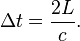$\Delta t = \frac{2 L}{c}.$
From the frame of reference of a moving observer traveling at the speed v (diagram at lower right), the light pulse traces out a longer, angled path. The second postulate of special relativity states that the speed of light is constant in all frames, which implies a lengthening of the period of this clock from the moving observer's perspective. That is to say, in a frame moving relative to the clock, the clock appears to be running more slowly. Straightforward application of the Pythagorean theorem leads to the well-known prediction of special relativity:

The total time for the light pulse to trace its path is given by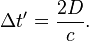$\Delta t' = \frac{2 D}{c}.$
The length of the half path can be calculated as a function of known quantities as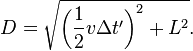$D = \sqrt{\left (\frac{1}{2}v \Delta t'\right )^2+L^2}.$
Substituting D from this equation into the previous and solving for Δt' gives: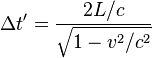$\Delta t' = \frac{2L/c}{\sqrt{1-v^2/c^2}}$
and thus, with the definition of Δt: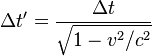$\Delta t' = \frac{\Delta t}{\sqrt{1-v^2/c^2}}$
which expresses the fact that for the moving observer the period of the clock is longer than in the frame of the clock itself.

## Time dilation due to relative velocity symmetric between observers

Common sense would dictate that if time passage has slowed for a moving object, the moving object would observe the external world to be correspondingly "sped up". Counterintuitively, special relativity predicts the opposite.

A similar oddity occurs in everyday life. If Sam sees Abigail at a distance she appears small to him and at the same time Sam appears small to Abigail. Being very familiar with the effects of perspective, we see no mystery or a hint of a paradox in this situation.

One is accustomed to the notion of relativity with respect to distance: the distance from Los Angeles to New York is by convention the same as the distance from New York to Los Angeles. On the other hand, when speeds are considered, one thinks of an object as "actually" moving, overlooking that its motion is always relative to something else — to the stars, the ground or to oneself. If one object is moving with respect to another, the latter is moving with respect to the former and with equal relative speed.

In the special theory of relativity, a moving clock is found to be ticking slowly with respect to the observer's clock. If Sam and Abigail are on different trains in near-lightspeed relative motion, Sam measures (by all methods of measurement) clocks on Abigail's train to be running slowly and similarly, Abigail measures clocks on Sam's train to be running slowly.

Note that in all such attempts to establish "synchronization" within the reference system, the question of whether something happening at one location is in fact happening simultaneously with something happening elsewhere, is of key importance. Calculations are ultimately based on determining which events are simultaneous. Furthermore, establishing simultaneity of events separated in space necessarily requires transmission of information between locations, which by itself is an indication that the speed of light will enter the determination of simultaneity.

It is a natural and legitimate question to ask how, in detail, special relativity can be self-consistent if clock A is time-dilated with respect to clock B and clock B is also time-dilated with respect to clock A. It is by challenging the assumptions built into the common notion of simultaneity that logical consistency can be restored. Simultaneity is a relationship between an observer in a particular frame of reference and a set of events. By analogy, left and right are accepted to vary with the position of the observer, because they apply to a relationship. In a similar vein, Plato explained that up and down describe a relationship to the earth and one would not fall off at the antipodes.

Within the framework of the theory and its terminology there is a relativity of simultaneity that affects how the specified events are aligned with respect to each other by observers in relative motion. Because the pairs of putatively simultaneous moments are identified differently by different observers (as illustrated in the twin paradox article), each can treat the other clock as being the slow one without relativity being self-contradictory. This can be explained in many ways, some of which follow.

### Temporal coordinate systems and clock synchronization

In Relativity, temporal coordinate systems are set up using a procedure for synchronizing clocks, discussed by Poincaré (1900) in relation to Lorentz's local time (see relativity of simultaneity). It is now usually called the Einstein synchronization procedure, since it appeared in his 1905 paper.

An observer with a clock sends a light signal out at time t1 according to his clock. At a distant event, that light signal is reflected back to, and arrives back to the observer at time t2 according to his clock. Since the light travels the same path at the same rate going both out and back for the observer in this scenario, the coordinate time of the event of the light signal being reflected for the observer tE is tE = (t1 + t2) / 2. In this way, a single observer's clock can be used to define temporal coordinates which are good anywhere in the universe.

Symmetric time dilation occurs with respect to temporal coordinate systems set up in this manner. It is an effect where another clock is being viewed as running slowly by an observer. Observers do not consider their own clock time to be time-dilated, but may find that it is observed to be time-dilated in another coordinate system.

## Overview of formulae

### Time dilation due to relative velocity

The formula for determining time dilation in special relativity is: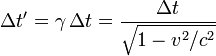$\Delta t' = \gamma \, \Delta t = \frac{\Delta t}{\sqrt{1-v^2/c^2}} \,$
where Δt is the time interval between two co-local events (i.e. happening at the same place) for an observer in some inertial frame (e.g. ticks on his clock) – this is known as the proper time, Δt ' is the time interval between those same events, as measured by another observer, inertially moving with velocity v with respect to the former observer, v is the relative velocity between the observer and the moving clock, c is the speed of light, and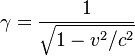$\gamma = \frac{1}{\sqrt{1-v^2/c^2}} \,$
is the Lorentz factor. Thus the duration of the clock cycle of a moving clock is found to be increased: it is measured to be "running slow". The range of such variances in ordinary life, where vc, even considering space travel, are not great enough to produce easily detectable time dilation effects and such vanishingly small effects can be safely ignored. It is only when an object approaches speeds on the order of 30,000 km/s (1/10 the speed of light) that time dilation becomes important.

Time dilation by the Lorentz factor was predicted by Joseph Larmor (1897), at least for electrons orbiting a nucleus. Thus "... individual electrons describe corresponding parts of their orbits in times shorter for the [rest] system in the ratio :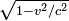$\sqrt{1 - v^2/c^2}$" (Larmor 1897). Time dilation of magnitude corresponding to this (Lorentz) factor has been experimentally confirmed, as described below.

### Time dilation due to gravitation and motion together

Astronomical time scales and the GPS system represent significant practical applications, presenting problems that call for consideration of the combined effects of mass and motion in producing time dilation.
Relativistic time dilation effects, for the solar system and the Earth, have been evaluated from the starting point of an approximation to the Schwarzschild solution to the Einstein field equations. A timelike interval dtE in this metric can be approximated, when expressed in rectangular coordinates and when truncated of higher powers in 1/c2, in the form: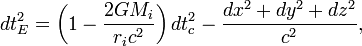$dt_E^2 = \left( 1-\frac{2GM_i}{r_i c^2} \right) dt_c^2 - \frac{dx^2+dy^2+dz^2}{c^2}, \,$
(1)
where:
dtE (expressed as a time-like interval) is a small increment forming part of an interval in the proper time tE (an interval that could be recorded on an atomic clock);
dtc is a small increment in the timelike coordinate tc ("coordinate time") of the clock's position in the chosen reference frame;
dx, dy and dz are small increments in three orthogonal space-like coordinates x, y, z of the clock's position in the chosen reference frame; and
GMi/ri represents a sum, to be designated U, of gravitational potentials due to the masses in the neighborhood, based on their distances ri from the clock. This sum of the GMi/ri is evaluated approximately, as a sum of Newtonian gravitational potentials (plus any tidal potentials considered), and is represented below as U (using the positive astronomical sign convention for gravitational potentials). The scope of the approximation may be extended to a case where U further includes effects of external masses other than the Mi, in the form of tidal gravitational potentials that prevail (due to the external masses) in a suitably small region of space around a point of the reference frame located somewhere in a gravity well due to those external masses, where the size of 'suitably small' remains to be investigated.
From this, after putting the velocity of the clock (in the coordinates of the chosen reference frame) as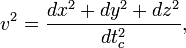$v^2=\frac{dx^2+dy^2+dz^2}{dt_c^2}, \,$
(2)
(then taking the square root and truncating after binomial expansion, neglecting terms beyond the first power in 1/c2), a relation between the rate of the proper time and the rate of the coordinate time can be obtained as the differential equation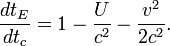$\frac{dt_E}{dt_c}= 1-\frac{U}{c^2}-\frac{v^2}{2c^2}. \,$
(3)
Equation (3) represents combined time dilations due to mass and motion, approximated to the first order in powers of 1/c2. The approximation can be applied to a number of the weak-field situations found around the Earth and in the solar-system. It can be thought of as relating the rate of proper time tE that can be measured by a clock, with the rate of a coordinate time tc.

In particular, for explanatory purposes, the time-dilation equation (3) provides a way of conceiving coordinate time, by showing that the rate of the clock would be exactly equal to the rate of the coordinate time if this "coordinate clock" could be situated
(a) hypothetically outside all relevant 'gravity wells', e.g. remote from all gravitational masses Mi, (so that U=0), and also
(b) at rest in relation to the chosen system of coordinates (so that v=0).
Equation (3) has been developed and integrated for the case where the reference frame is the solar system barycentric ('ssb') reference frame, to show the (time-varying) time dilation between the ssb coordinate time and local time at the Earth's surface: the main effects found included a mean time dilation of about 0.49 second per year (slower at the Earth's surface than for the ssb coordinate time), plus periodic modulation terms of which the largest has an annual period and an amplitude of about 1.66 millisecond.

Equation (3) has also been developed and integrated for the case of clocks at or near the Earth's surface. For clocks fixed to the rotating Earth's surface at mean sea level, regarded as a surface of the geoid, the sum ( U + v2/2 ) is a very nearly constant geopotential, and decreases with increasing height above sea level approximately as the product of the change in height and the gradient of the geopotential. This has been evaluated as a fractional increase in clock rate of about 1.1x10−13 per kilometer of height above sea level due to a decrease in combined rate of time dilation with increasing altitude. The value of dtE/dtc at height falls to be compared with the corresponding value at mean sea level. (Both values are slightly below 1, the value at height being a little larger (closer to 1) than the value at sea level.)

A fuller development of equation (3) for the near-Earth situation has been used to evaluate the combined time dilations relative to the Earth's surface experienced along the trajectories of satellites of the GPS global positioning system. The resulting values (in this case they are relativistic increases in the rate of the satellite-borne clocks, by about 38 microseconds per day) form the basis for adjustments essential for the functioning of the system.

This gravitational time dilation relationship has been used in the synchronization or correlation of atomic clocks used to implement and maintain the atomic time scale TAI, where the different clocks are located at different heights above sea level, and since 1977 have had their frequencies steered to compensate for the differences of rate with height.

In pulsar timing, the advance or retardation of the pulsar phase due to gravitational and motional time dilation is called the "Einstein Delay".

## Experimental confirmation

Time dilation has been tested a number of times. The routine work carried on in particle accelerators since the 1950s, such as those at CERN, is a continuously running test of the time dilation of special relativity. The specific experiments include:

### Velocity time dilation tests

• Ives and Stilwell (1938, 1941), "An experimental study of the rate of a moving clock", in two parts. The stated purpose of these experiments was to verify the time dilation effect, predicted by Lamor-Lorentz ether theory, due to motion through the ether using Einstein's suggestion that Doppler effect in canal rays would provide a suitable experiment. These experiments measured the Doppler shift of the radiation emitted from cathode rays, when viewed from directly in front and from directly behind. The high and low frequencies detected were not the classical values predicted.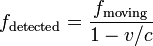$f_\mathrm{detected} = \frac{f_\mathrm{moving}}{1 - v/c}$ and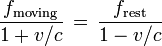$\frac{f_\mathrm{moving}}{1+v/c}\,=\, \frac{f_\mathrm{rest}}{1 - v/c}$ and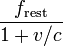$\frac{f_\mathrm{rest}}{1+v/c}$
i.e. for sources with invariant frequencies$f_\mathrm{moving}\, = f_\mathrm{rest}$ The high and low frequencies of the radiation from the moving sources were measured as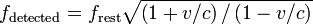$f_\mathrm{detected} = f_\mathrm{rest}\sqrt{\left(1 + v/c\right)/\left(1 - v/c\right) }$ and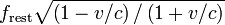$f_\mathrm{rest}\sqrt{\left(1 - v/c\right)/\left(1 + v/c\right)}$
as deduced by Einstein (1905) from the Lorentz transformation, when the source is running slow by the Lorentz factor.
• Rossi and Hall (1941) compared the population of cosmic-ray-produced muons at the top of a mountain to that observed at sea level. Although the travel time for the muons from the top of the mountain to the base is several muon half-lives, the muon sample at the base was only moderately reduced. This is explained by the time dilation attributed to their high speed relative to the experimenters. That is to say, the muons were decaying about 10 times slower than if they were at rest with respect to the experimenters.
• Hasselkamp, Mondry, and Scharmann (1979) measured the Doppler shift from a source moving at right angles to the line of sight (the transverse Doppler shift). The most general relationship between frequencies of the radiation from the moving sources is given by: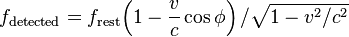$f_\mathrm{detected} = f_\mathrm{rest}{\left(1 - \frac{v}{c} \cos\phi\right)/\sqrt{1 - {v^2}/{c^2}} }$
as deduced by Einstein (1905). For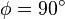$\phi = 90^\circ$ (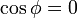$\cos\phi = 0\,$) this reduces to fdetected = frestγ. Thus there is no transverse Doppler shift, and the lower frequency of the moving source can be attributed to the time dilation effect alone.
• In 2010 time dilation was observed at speeds of less than 10 meters per second using optical atomic clocks connected by 75 meters of optical fiber.

### Gravitational time dilation tests

• Pound, Rebka in 1959 measured the very slight gravitational red shift in the frequency of light emitted at a lower height, where Earth's gravitational field is relatively more intense. The results were within 10% of the predictions of general relativity. Later Pound and Snider (in 1964) derived an even closer result of 1%. This effect is as predicted by gravitational time dilation.
• In 2010 gravitational time dilation was measured at the Earth's surface with a height difference of only one meter, using optical atomic clocks.

### Velocity and gravitational time dilation combined-effect tests

• Hafele and Keating, in 1971, flew caesium atomic clocks east and west around the Earth in commercial airliners, to compare the elapsed time against that of a clock that remained at the US Naval Observatory. Two opposite effects came into play. The clocks were expected to age more quickly (show a larger elapsed time) than the reference clock, since they were in a higher (weaker) gravitational potential for most of the trip (c.f. Pound, Rebka). But also, contrastingly, the moving clocks were expected to age more slowly because of the speed of their travel. The gravitational effect was the larger, and the clocks suffered a net gain in elapsed time. To within experimental error, the net gain was consistent with the difference between the predicted gravitational gain and the predicted velocity time loss. In 2005, the National Physical Laboratory in the United Kingdom reported their limited replication of this experiment. The NPL experiment differed from the original in that the caesium clocks were sent on a shorter trip (London–Washington D.C. return), but the clocks were more accurate. The reported results are within 4% of the predictions of relativity.
• The Global Positioning System can be considered a continuously operating experiment in both special and general relativity. The in-orbit clocks are corrected for both special and general relativistic time dilation effects as described above, so that (as observed from the Earth's surface) they run at the same rate as clocks on the surface of the Earth. In addition, but not directly time dilation related, general relativistic correction terms are built into the model of motion that the satellites broadcast to receivers — uncorrected, these effects would result in an approximately 7-metre (23 ft) oscillation in the pseudo-ranges measured by a receiver over a cycle of 12 hours.

A comparison of muon lifetimes at different speeds is possible. In the laboratory, slow muons are produced, and in the atmosphere very fast moving muons are introduced by cosmic rays. Taking the muon lifetime at rest as the laboratory value of 2.22 μs, the lifetime of a cosmic ray produced muon traveling at 98% of the speed of light is about five times longer, in agreement with observations. In this experiment the "clock" is the time taken by processes leading to muon decay, and these processes take place in the moving muon at its own "clock rate", which is much slower than the laboratory clock.

## Time dilation and space flight

Time dilation would make it possible for passengers in a fast-moving vehicle to travel further into the future while aging very little, in that their great speed slows down the rate of passage of on-board time. That is, the ship's clock (and according to relativity, any human travelling with it) shows less elapsed time than the clocks of observers on Earth. For sufficiently high speeds the effect is dramatic. For example, one year of travel might correspond to ten years at home. Indeed, a constant 1 g acceleration would permit humans to travel as far as light has been able to travel since the big bang (some 13.7 billion light years) in one human lifetime. The space travellers could return to Earth billions of years in the future. A scenario based on this idea was presented in the novel Planet of the Apes by Pierre Boulle.

A more likely use of this effect would be to enable humans to travel to nearby stars without spending their entire lives aboard the ship. However, any such application of time dilation during Interstellar travel would require the use of some new, advanced method of propulsion. The Orion Project has been the only major attempt toward this idea.

Current space flight technology has fundamental theoretical limits based on the practical problem that an increasing amount of energy is required for propulsion as a craft approaches the speed of light. The likelihood of collision with small space debris and other particulate material is another practical limitation. At the velocities presently attained, however, time dilation is not a factor in space travel. Travel to regions of space-time where gravitational time dilation is taking place, such as within the gravitational field of a black hole but outside the event horizon (perhaps on a hyperbolic trajectory exiting the field), could also yield results consistent with present theory.

### Time dilation at constant acceleration

In special relativity, time dilation is most simply described in circumstances where relative velocity is unchanging. Nevertheless, the Lorentz equations allow one to calculate proper time and movement in space for the simple case of a spaceship whose acceleration, relative to some referent object in uniform (i.e. constant velocity) motion, equals g throughout the period of measurement.

Let t be the time in an inertial frame subsequently called the rest frame. Let x be a spatial coordinate, and let the direction of the constant acceleration as well as the spaceship's velocity (relative to the rest frame) be parallel to the x-axis. Assuming the spaceship's position at time t = 0 being x = 0 and the velocity being v0 and defining the following abbreviation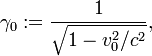$\gamma_0 := \frac{1}{\sqrt{1-v_0^2/c^2}},$
the following formulas hold:
Position: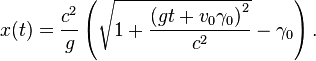$x(t) = \frac {c^2}{g} \left( \sqrt{1 + \frac{\left(gt + v_0\gamma_0\right)^2}{c^2}} -\gamma_0 \right).$
Velocity: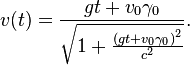$v(t) =\frac{gt + v_0\gamma_0}{\sqrt{1 + \frac{ \left(gt + v_0\gamma_0\right)^2}{c^2}}}.$
Proper time: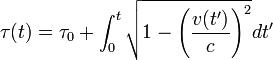$\tau(t) = \tau_0 + \int_0^t \sqrt{ 1 - \left( \frac{v(t')}{c} \right)^2 } dt'$
In the case where v(0) = v0 = 0 and τ(0) = τ0 = 0 the integral can be expressed as a logarithmic function or, equivalently, as an inverse hyperbolic function: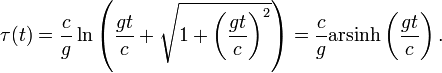$\tau(t) = \frac{c}{g} \ln \left( \frac{gt}{c} + \sqrt{ 1 + \left( \frac{gt}{c} \right)^2 } \right) = \frac{c}{g} \operatorname {arsinh} \left( \frac{gt}{c} \right) .$

### Spacetime geometry of velocity time dilation

The green dots and red dots in the animation represent spaceships. The ships of the green fleet have no velocity relative to each other, so for the clocks onboard the individual ships the same amount of time elapses relative to each other, and they can set up a procedure to maintain a synchronized standard fleet time. The ships of the "red fleet" are moving with a velocity of 0.866 of the speed of light with respect to the green fleet.
The blue dots represent pulses of light. One cycle of light-pulses between two green ships takes two seconds of "green time", one second for each leg.

As seen from the perspective of the reds, the transit time of the light pulses they exchange among each other is one second of "red time" for each leg. As seen from the perspective of the greens, the red ships' cycle of exchanging light pulses travels a diagonal path that is two light-seconds long. (As seen from the green perspective the reds travel 1.73 (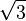$\sqrt{3}$) light-seconds of distance for every two seconds of green time.)
One of the red ships emits a light pulse towards the greens every second of red time. These pulses are received by ships of the green fleet with two-second intervals as measured in green time. Not shown in the animation is that all aspects of physics are proportionally involved. The light pulses that are emitted by the reds at a particular frequency as measured in red time are received at a lower frequency as measured by the detectors of the green fleet that measure against green time, and vice versa.

The animation cycles between the green perspective and the red perspective, to emphasize the symmetry. As there is no such thing as absolute motion in relativity (as is also the case for Newtonian mechanics), both the green and the red fleet are entitled to consider themselves motionless in their own frame of reference.
Again, it is vital to understand that the results of these interactions and calculations reflect the real state of the ships as it emerges from their situation of relative motion. It is not a mere quirk of the method of measurement or communication.

## References

1. ^ For sources on special relativistic time dilation, see Albert Einstein's own popular exposition, published in English translation (1920) as "Relativity: The Special and General Theory", especially at "8: On the Idea of Time in Physics", and in following sections 9–12. See also the articles Special relativity, Lorentz transformation and Relativity of simultaneity.
2. ^ Cassidy, David C.; Holton, Gerald James; Rutherford, Floyd James (2002), Understanding Physics, Springer-Verlag New York, Inc, ISBN 0-387-98756-8 , Chapter 9 §9.6, p. 422
3. ^ Cutner, Mark Leslie (2003), Astronomy, A Physical Perspective, Cambridge University Press, ISBN 0-521-82196-7 , Chapter 7 §7.2, p. 128
4. ^ Lerner, Lawrence S. (1996), Physics for Scientists and Engineers, Volume 2, Jones and Bertlett Publishers, Inc, ISBN 0-7637-0460-1 , Chapter 38 §38.4, p. 1051,1052
5. ^ Ellis, George F. R.; Williams, Ruth M. (2000), Flat and Curved Space-times, Second Edition, Oxford University Press Inc, New York, ISBN 0-19-850657-0 , Chapter 3 §1.3, p. 28-29
6. ^ Adams, Steve (1997), Relativity: an introduction to space-time physics, CRC Press, p. 54, ISBN 0-748-40621-2 , Section 2.5, page 54
7. ^ See T D Moyer (1981a), "Transformation from proper time on Earth to coordinate time in solar system barycentric space-time frame of reference", Celestial Mechanics 23 (1981) pages 33-56, equations 2 and 3 at pages 35-6 combined here and divided throughout by c2.
8. ^ A version of the same relationship can also be seen in Neil Ashby (2002), "Relativity and the Global Positioning System", Physics Today (May 2002), at equation (2).
9. ^ Such tidal effects can also be seen included in some of the relations shown in Neil Ashby (2002), cited above.
10. ^ (This is equation (6) at page 36 of T D Moyer (1981a), cited above.)
11. ^ G M Clemence & V Szebehely, "Annual variation of an atomic clock", Astronomical Journal, Vol.72 (1967), p.1324-6.
12. ^ T D Moyer (1981b), "Transformation from proper time on Earth to coordinate time in solar system barycentric space-time frame of reference" (Part 2), Celestial Mechanics 23 (1981) pages 57-68.
13. ^ J B Thomas (1975), "Reformulation of the relativistic conversion between coordinate time and atomic time", Astronomical Journal, vol.80, May 1975, p.405-411.
14. ^ See Neil Ashby (2002), cited above; also in article Global Positioning System the section Special and general relativity and further sources cited there.
15. ^ B Guinot (2000), "History of the Bureau International de l'Heure", ASP Conference Proceedings vol.208 (2000), pp.175-184, at p.182.
16. ^ "Journal Article". SpringerLink. Retrieved 2009-10-18.
17. ^ a b Chou, C. W.; Hume, D. B.; Rosenband, T.; Wineland, D. J. (2010). "Optical Clocks and Relativity". Science 329: 1630. doi:10.1126/science.1192720.  edit
19. ^ JV Stewart (2001), Intermediate electromagnetic theory, Singapore: World Scientific, p. 705, ISBN 9810244703
20. ^   (Equations (3), (4), (6), (9) on pages 5-6)
• Callender, Craig & Edney, Ralph (2001), Introducing Time, Icon, ISBN 1-84046-592-1
• Einstein, A. (1905) "Zur Elektrodynamik bewegter Körper", Annalen der Physik, 17, 891. English translation: On the electrodynamics of moving bodies
• Einstein, A. (1907) "Über eine Möglichkeit einer Prüfung des Relativitätsprinzips", Annalen der Physik.
• Hasselkamp, D., Mondry, E. and Scharmann, A. (1979) "Direct Observation of the Transversal Doppler-Shift", Z. Physik A 289, 151–155
• Ives, H. E. and Stilwell, G. R. (1938), "An experimental study of the rate of a moving clock", J. Opt. Soc. Am, 28, 215–226
• Ives, H. E. and Stilwell, G. R. (1941), "An experimental study of the rate of a moving clock. II", J. Opt. Soc. Am, 31, 369–374
• Joos, G. (1959) Lehrbuch der Theoretischen Physik, 11. Auflage, Leipzig; Zweites Buch, Sechstes Kapitel, § 4: Bewegte Bezugssysteme in der Akustik. Der Doppler-Effekt.
• Larmor, J. (1897) "On a dynamical theory of the electric and luminiferous medium", Phil. Trans. Roy. Soc. 190, 205–300 (third and last in a series of papers with the same name).
• Poincaré, H. (1900) "La theorie de Lorentz et la Principe de Reaction", Archives Neerlandaies, V, 253–78.
• Reinhardt et al. Test of relativistic time dilation with fast optical atomic clocks at different velocities (Nature 2007)
• Rossi, B and Hall, D. B. Phys. Rev., 59, 223 (1941).
• NIST Two way time transfer for satellites
• Voigt, W. "Ueber das Doppler'sche princip" Nachrichten von der Königlicher Gesellschaft der Wissenschaften zu Göttingen, 2, 41–51.